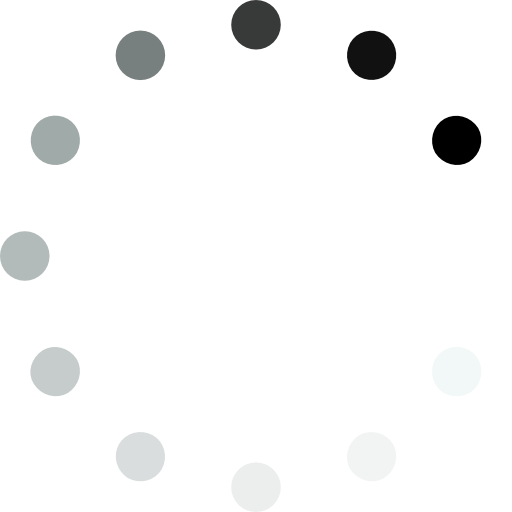### Dobble

Dobble is a monomatch game where you need to find the matching symbol in the two cards that are shown. Every card contains a symbol that will match with a symbol on any other card. Check out the official game here!

### The algorithm

```                ```

//order of plane, must be a prime number
let n = 7

//order of plane + 1
let numOfSymbols = n + 1

//the deck of cards
let cards = []

function createDeck(){
let i, j, k;

//the current card we are building
let card = [];

//to start, we build the first card
for (i = 1; i<= n+1; i++) {
card.push(i)
}

cards.push(card)

//then we build the next n number of cards
for (j=1; j<=n; j++) {
card = []
card.push(1)

for (k=1; k<=n; k++) {
card.push(n * j + (k+1))
}
cards.push(card)
}

//finally we build the next n² number of cards
for (i= 1; i<=n; i++) {
for (j=1; j<=n; j++) {
card = []
card.push(i+1)

for (k=1; k<= n; k++) {
card.push(n+2+n*(k-1)+(((i-1)*(k-1)+j-1)%n))
}
cards.push(card)
}
}
```
```